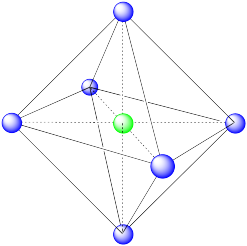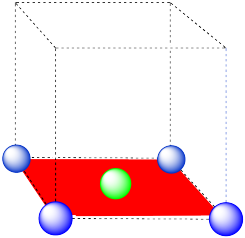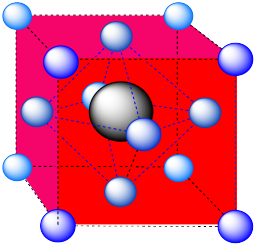S. Solutions to Selected Problems

Problem ME1.1.

The second metal atom is a different size than the principle metal atom. It will not quite fit into the array of atoms. Consequently, the atoms will not be able to slide past each other as easily.

Problem ME1.2.

2 Fe: (3+) x2 = (6+)

3 O: (2-) x3 = (6-)

Fe2O3: neutral

Problem ME1.3.

The longer the box, the longer the possible wavelength.

Problem ME2.1.

The pattern also repeats diagonally.

Problem ME2.2.

The squares can form a number of regularly repeating patterns. The rows can exactly repeat (an aaa pattern), or they can be shifted slightly, so that every other row exactly repeats the first row (an ababab pattern). They could even be shifted so that every third row is an exact repeat of the first (abcabcabc).

The pentagons do not form a repeating layer in two dimensions.

Problem ME2.3.

In the simple square packing, the holes are roughly diamond shaped.

In the hexagonal pattern, the holes are roughly triangular.

Problem ME2.6.

1. Using quarters, the distance is about 5 mm.
2. Using quarters, the distance is about 2.5 mm.

A free electron would be closer to the atoms in a hexagonal close packed layer. There would be stronger electron-ion attraction in that case.

Problem ME2.7.

1. Using dimes, the distance is about 3.5 mm.
2. Using dimes, the distance is about 1.5 mm.

A free electron would be closer to the atoms if the atoms were smaller. There would be stronger electron-ion attraction in that case.

Problem ME2.8.

1. melting points (and force of attraction between atoms): Fr < Cs < K < Na < Li
2. This trend mirrors the sizes of the atoms. Lithium is the smallest and francium the largest. The electron / ion attraction is greatest in Li and weakest in Fr.

Problem ME2.9.

a) The total area of this square is

Area = w2

in which w = width of the unit cell. The width of the unit cell is

w = 2r

in which r = radius of titanium atom.

Area = 4r2 = 4 (2.00 x 10-10 m)2 = 4(4.00 x 10-20 m2) = 1.60 x 10-19 m2

b) The total area of this rhombus is

Area = s2 sin θ

in which s = one side of unit cell and θ = an angle of the unit cell (either 60° or 120°). But

s = 2r

in which r = radius of titanium atom.

Area = (2 x 2.00 x 10-10 m)2 sin (60) = 1.60 x 10-19 (0.87) m2 = 1.39 x 10-19 m2

Problem ME2.10

1. 4 x 1/4 = 1 atom
2. 2 x 1/6 + 2 x 1/3 = 2/6 + 4/6 = 1 atom

Problem ME2.11.

The cross-sectional (two dimensional) area of a titanium atom is the area of a circle

Area = π r2

Area = 3.1415 x (2.00 x 10-10 m)2 = 3.1415 x 4.00 x 10-20 m2 = 1.257 x 10-19 m2 = 1.26 x 10-19 m2

Problem ME2.12.

a) Efficiency = (occupied / total) x 100 % = (1.26 x 10-19 m2 / 1.60 x 10-19 m2) x 100% = 79%

b) Efficiency = (occupied / total) x 100 % = (1.26 x 10-19 m2 / 1.39 x 10-19 m2) x 100% = 91%

A greater percentage of space is occupied in hexagonal close packing.

Problem ME2.13.

1. coordination number = 4
2. coordination number = 6

Problem ME2.14.

1. Assuming the pattern extends linearly, coordination number = 2. Otherwise, coordination number = 2 for interior atoms and coordination number = 1 for terminal atoms.
2. Assuming the pattern extends in two dimensions, coordination number = 3. Otherwise, coordination number = 3 for interior atoms, coordination number = 2 for side edge atoms and coordination number = 1 for corner atoms.

Problem ME3.1.

1. 1/8 atom at each corner
2. 8 corners in a cube
3. 8 x 1/8 = 1 atom per simple cube

Problem ME3.2.

1. 1/8 atom at each corner
2. 8 corners in a cube
3. 1 atom in middle of cube
4. 1 + 8 x 1/8 = 2 atoms per body-centered cube

Problem ME3.3.

Hexagonal close packed pattern is ABAB.

Problem ME3.4.

1. At both the top and bottom layer, two atoms are 1/3 within the cell and two atoms are 1/6 within the cell.
2. That makes 2 x ( 2 x 1/3 + 2 x 1/6) = 4/3 + 4/6 = 4/3 + 2/3 = 1 atom total in corners
3. 1 atom inside the cell.
4. 2 atoms total.

Problem ME3.5.

The pattern in this variation is ABACA.

ABCABC.

Problem ME3.7.

1. The rhombus-shaped hexagonal unit cell.
2. 4 layers.

Problem ME3.8.

1. 1/8 atom at each corner
2. 8 corners in a cube
3. 1/2 atom on each face of cube
4. 6 x 1/2 + 8 x 1/8 = 4 atoms per face-centered cube

Problem ME3.9.

a) coordination number = 6 (3 within the cube, along the three adjacent edges, plus three on adjacent cubes).

In the picture, consider the green atom coordinated by its nearest neighbours, the blue atoms. One unit cell is highlighted in red.b) coordination number = 8 (corners of the cube).A single unit cell is shown below for simplicity.c) the closest atoms are in the centers of the adjacent cells; the other corners are further away; coordination number = 8.A single unit cell is shown below, for simplicity.d) The closest atoms are on the faces adjacent to the corner position; these faces are found along three planes, with four faces surrounding the corner on each plane; coordination umber = 12.A single unit cell is shown below.e) The closest atoms are at the corners around the face; coordination number = 4.A single unit cell is shown below.Problem ME3.10.

a) Octahedral. An octahedron has six vertices and eight faces.b) Cubic. A cube has eight vertices and six faces.c) This is also a cube, translated from the last one (i.e. the cube is just shifted to a different position in the lattice).d) Similar to icosahedral, but distorted from ideal icosahedral geometry. An icosahedron has 12 vertices and 20 faces. This shape has some pairs of faces flattened out together, and so it has only 14 faces total.e) Square planar. The central atom is in the middle of a square formed by four other atoms.Problem ME3.11.

a) A cubic hole.b) A square pyramidal hole. The atom in the hole is above a square of atoms, with an additional atom right above it.c) An octahedral hole. The atom occupying the hole has been pushed all the way to the face of the cube. It is right in the middle of a square of atoms. One of the vertices is in the center of the next cube.d) A tetrahedral hole. Note that the layers in the cubic closest-packed system run diagonally through the cubic unit cell. The atom in the hole sits above a trio of atoms on three adjacent faces, and is topped by the atom in the corner of the cube. Because a tetrahedron is so symmetric, the arrangement can be described in a number of other ways, choosing any three surrounding atoms as the base and the fourth atom as the cap of the tetrahedron.e) An octahedron. Again, the hexagonal layers run diagonally through the unit cell.